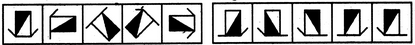# Non Verbal Reasoning - Series - Discussion

Discussion Forum : Series - Section 1 (Q.No. 38)
Directions to Solve

Each of the following questions consists of five figures marked A, B, C, D and E called the Problem Figures followed by five other figures marked 1, 2, 3, 4 and 5 called the Answer Figures. Select a figure from amongst the Answer Figures which will continue the same series as established by the five Problem Figures.

38.

Select a figure from amongst the Answer Figures which will continue the same series as established by the five Problem Figures.(A)     (B)     (C)     (D)     (E)       (1)     (2)     (3)     (4)     (5)
1
2
3
4
5
Explanation:
Similar figure reappears in every fourth step and each time a figure reappears, it rotates through 90oACW.
Discussion:
2 comments Page 1 of 1.

Aashi said:   6 years ago
(A) and (E) are similar figures rotated through 90 degrees so to find out the answer, (B) has to be rotated through,

90 degree and thus the answer is option 3.

Ajay Kumar said:   10 years ago
I do not understand that similar figure reappears in every fourth step and each time a feature reappears. Please explain detail?